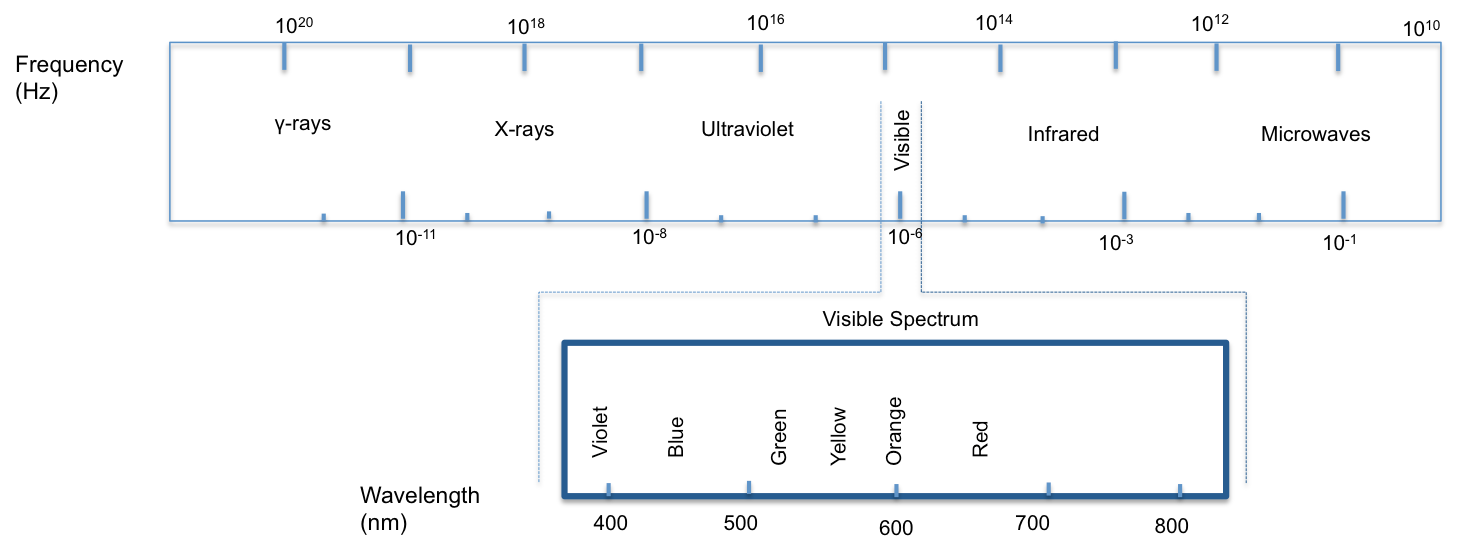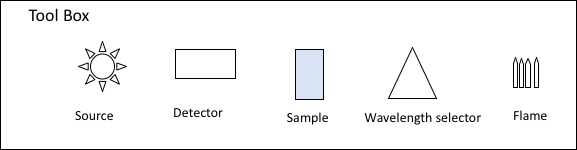# Comparing Spectroscopic Techniques

1. Explain molecular origins and fundamental equations of spectroscopy. And illustrate energy diagrams for spectroscopic techniques (UV-Vis, FL, AA).
1. The image below illustrates the Electromagnetic Spectrum.What is the relationship between the energy, frequency, & wavelength of electromagnetic radiation?

2. Draw energy diagrams (Jablonski diagrams) for the techniques listed. Indicated ABSORPTION, FLUORESCENCE, or PHOPHORESCENCE energy transitions as needed.  Use appropriate arrow notation (straight line = radiative process, wavy line = non-radiative process).3. On one chart, crudely sketch both an excitation (EX) spectrum and an emission (EM) spectrum for the same fluorescing molecule.4. What factors influence the absorbance that you would measure for a sample? Is each factor directly or inversely proportional to the absorbance? Hint: Beer’s Law

1. Sketch instrumental schematics for each spectrophotometer (UV-Vis, FL, AA).
1. On your white board, draw a simple block diagram for each type of spectrometer (UV-Vis, FL, AA) outlined below. Each block diagram should include sources, wavelength selectors, detectors, sample and path of radiation. Use the tool box for help.1. Instrument disassembly/analysis of a UV-Vis. The parts listed below will be addressed.
• slit width
• filters
• Concave mirrors
• monochromator
• Beam splitter
• two detectors
• Sample holder
• Double beam Spec difference
• UV-Vis vs. Fluorometer
• Length of spec
• Black interior
1. Analysis of unknown samples A, B, & C using UV-Vis, FL, AA.
1. Scan all three samples in each of the instruments and note if spectra appears, to what extent, and at what wavelength.

Sample

A

B

C

UV-Vis

Peak Present:

λ max =

Peak Shape:

Peak Present:

λ max =

Peak Shape:

Peak Present:

λ max =

Peak Shape:

FL

λ Ex =

λ Em =

Peak Intensity

λ Ex =

λ Em =

Peak Intensity

λ Ex =

λ Em =

Peak Intensity

AA

λ Abs =

Absorption: Yes |No

λ Abs =

Absorption: Yes |No

λ Abs =

Absorption: Yes |No

2. Three possible identities for the unknown compounds are listed below. Identify which one of them would be compound A, B, and C.

1. Interrogate compound classification using three spectroscopic techniques (UV-Vis, FL, AA) and select appropriate technique.
1. Which technique would be optimal for analysis of the following compounds? Justify your answers.